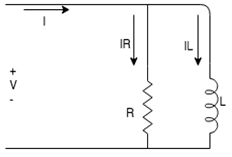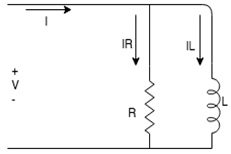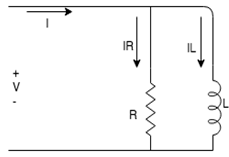Test: Parallel Impedance Circuits

# Test: Parallel Impedance Circuits

Test Description

## 10 Questions MCQ Test Basic Electrical Technology | Test: Parallel Impedance Circuits

Test: Parallel Impedance Circuits for Electrical Engineering (EE) 2023 is part of Basic Electrical Technology preparation. The Test: Parallel Impedance Circuits questions and answers have been prepared according to the Electrical Engineering (EE) exam syllabus.The Test: Parallel Impedance Circuits MCQs are made for Electrical Engineering (EE) 2023 Exam. Find important definitions, questions, notes, meanings, examples, exercises, MCQs and online tests for Test: Parallel Impedance Circuits below.
Solutions of Test: Parallel Impedance Circuits questions in English are available as part of our Basic Electrical Technology for Electrical Engineering (EE) & Test: Parallel Impedance Circuits solutions in Hindi for Basic Electrical Technology course. Download more important topics, notes, lectures and mock test series for Electrical Engineering (EE) Exam by signing up for free. Attempt Test: Parallel Impedance Circuits | 10 questions in 10 minutes | Mock test for Electrical Engineering (EE) preparation | Free important questions MCQ to study Basic Electrical Technology for Electrical Engineering (EE) Exam | Download free PDF with solutions
 1 Crore+ students have signed up on EduRev. Have you?
Test: Parallel Impedance Circuits - Question 1

### In an impedance parallel network, the reactive component will ____________ the voltage by 90 degrees.

Detailed Solution for Test: Parallel Impedance Circuits - Question 1

In an impedance parallel network the reactive component will either lead or lag the voltage by 90 degrees.

Test: Parallel Impedance Circuits - Question 2

### In an impedance parallel network the reactive component will either lead or lag the voltage by _________ degrees.

Detailed Solution for Test: Parallel Impedance Circuits - Question 2

In an impedance parallel network the reactive component will either lead or lag the voltage by 90 degrees.

Test: Parallel Impedance Circuits - Question 3

### In an impedance parallel network the reactive component will either lead or lag the ________ by 90 degrees.

Detailed Solution for Test: Parallel Impedance Circuits - Question 3

In an impedance parallel network the reactive component will either lead or lag the voltage by 90 degrees.

Test: Parallel Impedance Circuits - Question 4

The reactive component in an impedance parallel circuit leads the voltage when the current _________ the voltage.

Detailed Solution for Test: Parallel Impedance Circuits - Question 4

The reactive component in an impedance parallel circuit leads the voltage when the current leads the voltage.

Test: Parallel Impedance Circuits - Question 5

The phase difference between the active component of an impedance parallel circuit and the voltage in the network is __________

Detailed Solution for Test: Parallel Impedance Circuits - Question 5

The active component in an impedance parallel network will always be in phase with the voltage in the circuit. Hence the phase difference is 0.

Test: Parallel Impedance Circuits - Question 6

The quadrature component is also known as?

Detailed Solution for Test: Parallel Impedance Circuits - Question 6

The quadrature component is also known as the reactive component because the reactive component forms a quadrature with the voltage.

Test: Parallel Impedance Circuits - Question 7

The quadrature component is also known as?

Detailed Solution for Test: Parallel Impedance Circuits - Question 7

The quadrature component is also known as the reactive component because the reactive component forms a quadrature with the voltage.

Test: Parallel Impedance Circuits - Question 8

What is the phase relation between IL and V from the following circuit?Detailed Solution for Test: Parallel Impedance Circuits - Question 8

IL is the current across the inductor and we know that the current across the inductor always lags the voltage across it. Hence IL lags V.

Test: Parallel Impedance Circuits - Question 9

What is the expression for the current in the inductor from the following circuit?Detailed Solution for Test: Parallel Impedance Circuits - Question 9

In the given circuit, the voltage across the inductor is the same as the source voltage as they are connected in parallel. The current in the inductor is IL hence IL=V/XL.

Test: Parallel Impedance Circuits - Question 10

Find the total current if IL=2A and Ir=8A.​Detailed Solution for Test: Parallel Impedance Circuits - Question 10

We know that I=IR+IL.
Substituting the values from the question, we get I=10A.

## Basic Electrical Technology

58 docs|59 tests
 Use Code STAYHOME200 and get INR 200 additional OFF Use Coupon Code
Information about Test: Parallel Impedance Circuits Page
In this test you can find the Exam questions for Test: Parallel Impedance Circuits solved & explained in the simplest way possible. Besides giving Questions and answers for Test: Parallel Impedance Circuits, EduRev gives you an ample number of Online tests for practice

58 docs|59 tests